Mobile QR Code1. (Department of Electronic and Electrical Engineering, POSTECH (Pohang University of Science and Technology), Pohang 37673, Korea )
2. (Samsung Electronics, Hwaseong 445-701, Korea)

Low power interface, short-reach interface, relaxed termination, time-based receiver, IIR DFE, IIR filter

## I. INTRODUCTION

Demands for low-power high bandwidth interface between DRAM and processor keep on increasing with the advent of cloud computers and deep-learning. Recently, a high-bandwidth memory (HBM) was introduced to meet these demands (1). The HBM increases the memory bandwidth by using hundreds of parallel data lines and the data-rate of single data line is a few Gb/s. The through-silicon-via (TSV) technology is used in HBM to build a short-reach interconnect with small capacitance loading for each data line. No termination resistance is used in HBM to reduce the signaling power of transmitter (TX) driver.

However, because the HBM technology is costly, a short-reach PCB interconnect between DRAM and ASIC chips can be used to implement an inexpensive deep-learning accelerator; a DRAM chip is placed on one side of printed circuit board (PCB) and an ASIC chip is placed on the opposite side of PCB and then the PCB with two chips is placed in a package for system-in-package (SIP) application (2). This work was initiated to implement a short-reach PCB interconnect economically without using HBM. Only CMOS inverters are used for transmitter and receiver in HBM because of very small capacitance of TSV. However, a larger capacitance is associated with the short-reach PCB interconnect of this work; the bonding-wire pad capacitance and the PCB transmission line capacitance contribute to the capacitance of the short-reach PCB interconnect. Because of the larger interconnect capacitance, this work requires termination and equalization, which are not necessary with HBM. The short-reach PCB interconnect can be used for general chip-to-chip interface on PCB if two signal pins to be connected are located very close from each other (Fig. 1(a)). When the interconnect length is much shorter than the wavelength of the highest signal frequency (0.35/TR), it can be approximated as RLC lumped circuit rather than as transmission line (3); TR is the 10%-to-90% signal rise time. The short-reach PCB interconnect can use on-die-termination (ODT) resistance which is much larger than 50 Ω; the ODT resistance at TX and receiver (RX) reduces the ringing due to the inductive component such as bonding wire. The short-reach PCB interconnect with ODT is modeled in Fig. 1(b). RTX and RRX represent the ODT resistance at TX and RX, respectively; total inductance (L) includes the inductance of interconnect channel (LCH) and bonding wire (LBOND), and total capacitance (C) includes the capacitance of interconnect channel (CCH) and chip pin (CPAD).

Fig. 1. (a) Short-reach interface implemented on PCB, (b) Lumped equivalent circuit of short-reach PCB interconnect.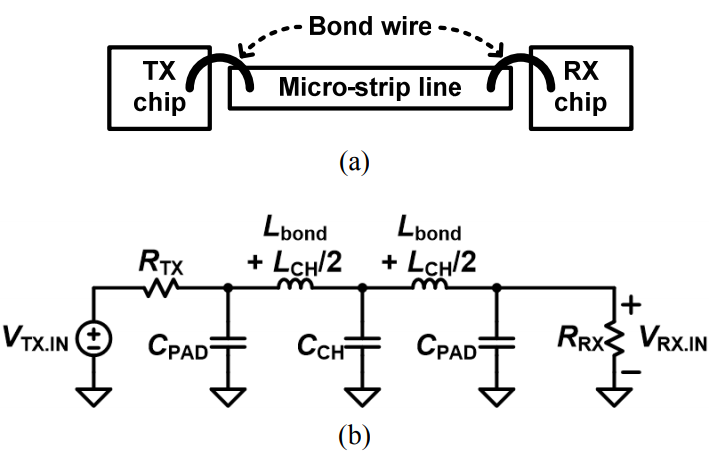The TX driver circuit (VTX.IN and RTX) in Fig. 1(b) is a voltage mode driver, which swings between 0 and VDD (supply voltage). As VDD is reduced, the TX power is reduced but the RX power is increased because the voltage swing at RX input is also reduced proportionally and hence a higher-gain RX circuit is required to recover digital data from the reduced-swing RX input. While the RX gain is high at high VDD in the conventional voltage-based circuits, it is high at low VDD in the time-based (TB) circuits (4); this is due to the voltage-to-time converter (VTC) used as the pre-amplifier of the TB RX circuit. The VTC gain is proportional to C/gm which increases as VDD is reduced. This property of VTC helps to reduce the TX power as well as the RX power in the TB circuits. Several TB RX circuits are published for low-power operation (4-6).

When the transfer function of interconnect is a single-time-constant equation, its single-bit response is an exponentially decaying waveform and hence its ISI can be compensated completely by a 1-tap IIR DFE. However, the single-bit response of the short-reach PCB interconnect (Fig. 1(a)) is a combination of an exponentially decaying waveform with single time constant and a ringing waveform. With the increase of RTX and RRX in Fig. 1(b), its single-bit response looks like an exponentially decaying waveform with small ringing terms. Besides, the large RTX and RRX reduces the TX power. In this work, a 1-tap IIR DFE is used at RX with large RTX and RRX.

Section II explains the transmission channel for the short-reach PCB interconnect used in this work. Section III describes the operating principle of the proposed TB IIR DFE circuit. Section IV presents the circuit implementation. Section V shows the measurement results. Section VI concludes this work.

## II. 1-TAP IIR DFE FOR SHORT-REACH PCB INTERCONNECT

To achieve a short-reach PCB interconnect, TX and RX chips are placed on a PCB by using a chip-on-board (COB) package technique; TX and RX chips are connected through a 1.6 mm micro-strip transmission line on the PCB (Fig. 1(a)). The 10%-to-90% rise time (TR) of the signal applied to the micro-strip line is ~ 60 ps; the highest frequency with significant energy is 5.83 GHz (0.35/TR) with the wavelength of 25.7 mm (3). Because the micro-strip line is shorter than one eighth the wavelength of the highest frequency (3.2 mm), the transmission channel can be approximated as a lumped circuit (Fig. 1(b)). RTX and RRX are the termination resistance of TX and RX, respectively. The capacitance (C) is 2.0 pF, which includes the chip pin capacitance (2×0.9 pF) and the micro-strip line capacitance (0.2 pF). The inductance (L) is 1 nH, which is the sum of the series inductance of two 0.5-mm long double bonding wires (0.5 nH) and the micro-strip line inductance (0.5 nH). The ratio of RTX to RRX is set to 3 to 1 to keep the VRX.IN swing between 0 and VDD/4. The small channel swing reduces the dynamic power consumption of TX driver, but requires a high-gain high-power RX front-end circuit in conventional transceiver circuits. In the time-based RX of this work, the RX front-end circuit (VTC: voltage-to-time converter) can achieve a high gain with low power compared to the conventional circuit, and consumes the same power independent of input voltage swing. Therefore, in this paper, the ratio of RTX and RRX was fixed at 3:1 to achieve 200 mV swing at VDD = 800 mV. Besides, RTX and RRX were increased together to reduce static power as well; RRX ranges from 80 Ω to 480 Ω in this work.

The short-reach PCB interconnect (Fig. 1(b)) can be approximated as a RC channel with single-time-constant of (RRX||RTX)·C; the L/(RRX+RTX) time constant is not included because it is much smaller than the RC time constant. Using this property of the short-reach PCB interconnect, a 1-tap IIR DFE was used in this work to compensate for ISI.

In this work, there are three limiting factors in increasing the data-rate. One is the linearity limit; the RX input eye opening must be located inside the linear input range of RX front-end circuit for proper DFE compensation. Another is the sensitivity limit; a non-zero eye opening is required at RX input. The other is the LC resonance limit; the data-rate must be lower than the LC resonance frequency of channel. To identify the three limiting factors, the channel single-bit response, ISI and the RX eye opening are derived in the following paragraphs.

The single-bit response of the short-reach PCB interconnect (Fig. 2) is a combination of an exponentially decaying term with single-time-constant and an exponentially decaying ringing term. The single-time-constant exponentially decaying term corresponds to the approximated RC channel and the ringing term is originated by the LC resonance at ~7.38 GHz. To verify the RC channel approximation, the channel single-bit response is compared between the original channel model including a 1.6 mm-long lossy transmission line model and the approximated RC channel (Fig. 2). A unit pulse is applied at TX; the rise and fall times are 60 ps, respectively. The single-bit response is used instead of the impulse response, to model the more realistic situation where the signals have non-zero rise and fall times. The red x is the single-bit response of VRX.IN for the original channel and the blue dot is the single-bit response of VRX.IN for the approximated RC channel in Fig. 2. Let G(z) be the single-bit response of the original channel and H(z) be the approximated single-bit response of the channel.

Fig. 2. Normalized single-bit response of original channel (red x) and approximated RC channel (blue dot) with (a) RRX = 240 Ω at 8 Gb/s, (b) RRX = 120 Ω at 10 Gb/s.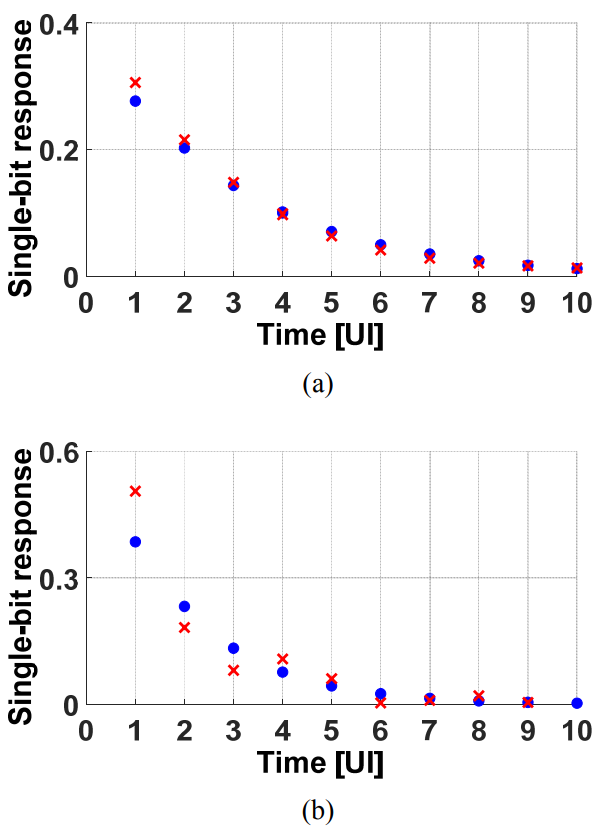##### (1)
$$\mathrm{G}(\mathrm{z})=g_{0}+g_{1} \cdot \mathrm{z}^{-1}+g_{2} \cdot \mathrm{z}^{-2}+g_{3} \cdot \mathrm{z}^{-3} \cdots$$

##### (2)
$$\mathrm{H}(\mathrm{z})=h_{0}+h_{1} \cdot \mathrm{z}^{-1}+h_{2} \cdot \mathrm{z}^{-2}+h_{3} \cdot \mathrm{z}^{-3} \cdots$$

Let gn be the time-domain single-bit response of the short-reach PCB interconnect channel. hn is the time-domain single-bit response of the channel with no inductance; hn is an exponentially decaying waveform with single time constant of (RTX||RRX)∙C. gn is a combination of hn and a ringing waveform. The difference between the real channel (gn) and the approximated channel (hn) is characterized by a ringing factor (fringing).

##### (3)
$$f_{\text {ringing }}=\sum_{\mathrm{n}=1}^{\infty}\left|g_{\mathrm{n}}-h_{\mathrm{n}}\right| / \sum_{\mathrm{n}=1}^{\infty}\left|g_{\mathrm{n}}\right|$$

Fig. 3. Contour plot of ringing factor (fringing, Eq. (3)) for different values of data-rate and RRX.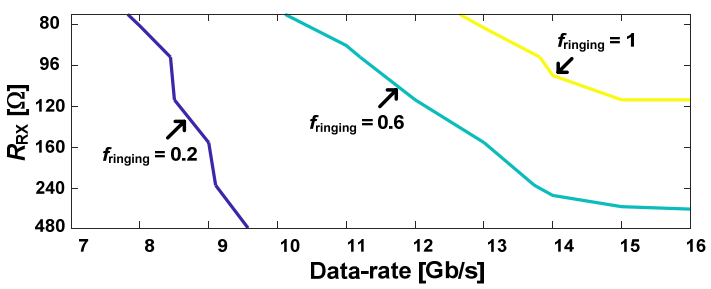fringing represents the remaining ISI ratio after the 1-tap IIR DFE operation. With the increase of RTX and RRX, fringing is reduced and gn approaches hn. fringing values were calculated from gn and hn values that are generated by simulation for different values of RRX and data-rate; RTX was set to 3∙RRX (Fig. 3). In the range of RRX > 120  and data-rate < 12 Gb/s, the transceiver of this paper was measured to work successfully with bit-error-rate < 10-12, where fringing < 0.6.

The worst case ISI after the 1-tap IIR DFE operation (ISIEQ) is the sum of absolute values of single-bit response errors from |g1 - h1| to |g - h|.

##### (4)
$$I S I_{\mathrm{EQ}}=\sum_{\mathrm{n}=1}^{\infty}\left|g_{\mathrm{n}}-h_{\mathrm{n}}\right|$$

Without equalization, the eye opening (EYE) of the RX input voltage (VRX.IN) is g0 - ISINOEQ (7); ISINOEQ is the worst case ISI which is the sum of absolute values of tail single-bit responses from |g1| to |g|. With the 1-tap IIR DFE, ISI is reduced to ISIEQ (Eq. (4)) and the RX eye is widened to g0 - ISIEQ.

##### (5)
$$E Y E_{\mathrm{EQ}}=g_{0}-\sum_{\mathrm{n}=1}^{\infty}\left|g_{\mathrm{n}}-h_{\mathrm{n}}\right|$$

To meet the linearity limit, the RX input voltage must be located within the normalized linear RX input range of [- 0.5·LR, 0.5·LR] in the entire normalized RX input range of [- 0.5, 0.5]; LR is a constant between 0 and 1. Due to the ISI of ‘0’s, the single-bit response (‘000∙∙∙0001’) rises from - 0.5, and g0 - 0.5 must be larger than - 0.5∙LR so that ‘1’ can be located within the linear RX input range. The linearity limit is stated by Eq. (6).

##### (6)
$$g_{0}>0.5-0.5 \cdot L R$$

To meet the sensitivity limit, the RX input eye must be larger than the RX input sensitivity (VRX.SENSITIVITY) to recover the correct digital data at RX output within 1 UI; the normalized VRX.SENSITIVITY is estimated to be 0.12 in this work.

##### (7)
$$E Y E_{\mathrm{EQ}}>V_{\mathrm{RX} . \text { SENSITIVTY }}$$

To meet the LC resonance limit, the data-rate must be much lower than twice the LC resonance frequency (7.38 GHz) to avoid a large ISI due to the ringing of channel single-bit pulse response gn.

## III. OPERATING PRINCIPLE OF TIME-BASED IIR DFE

The ISI of short-reach PCB interconnect is compensated by 1-tap IIR DFE because the short-reach PCB interconnect can be approximated as a single-time-constant (RC) channel if fringing is small. The loop delay of the IIR DFE is required to be < 1 UI in this work for efficient implementation. TB RX circuits are more suitable to include the IIR DFE than the voltage-based RX, because of the small loop delay; this is because the equalization operation is performed in the TB RX using simple digital circuits with small capacitive loading, such as inverters and NAND SR-latch (4). Because of the feedback loop delay in the 1-tap IIR DFE circuit, the 1st tap ISI (h1) cannot be compensated completely by the IIR DFE alone (8,9). As in Fig. 4, due to the feedback loop delay (Tdelay), at t = 1 T the RC-filtered voltage (VIIR) reaches h1.IIR which is smaller than h1. The remaining part (h1 ‒ h1.IIR) is compensated by a 1 tap FIR DFE (h1.FIR = h1 ‒ h1.IIR).

The TB RX is implemented by cascading VTC, TB DFE and time comparator (TCMP) (Fig. 5). The VTC converts the RX input voltage (VRX.IN) into a clock-like signal pair (CP, CN) which are two return-to-zero (RZ) signals with the rising edges separated by a time interval of TC; TC is defined to be the time interval from the rising edge of CP to the rising edge of CN that occurs after the falling edge of CK, and is proportional to (VRX.IN - VREF). For VRX.IN = ‘1’, the rising edge of CP comes before CN; for VRX.IN = ‘0’, the rising edge of CN comes before CP. ISI reduces |TC|, the time interval between the two rising edges. The TCMP recovers a voltage level RZ digital data (FP, FN) by identifying whose rising edge comes first. The equalization in the time-domain (TB FIR and IIR DFE) generates another clock-like RZ signal pair (OP, ON) such that the time interval (|TO|) between the rising edges of OP and ON is large enough for TCMP to easily identify ‘1’ or ‘0’; TO is defined to be the time interval from the rising edge of OP to the rising edge of ON that occurs after the falling edge of CK. This basic operation of TB RX is described in detail in (4). The TB DFE of this work consists of a cascaded connection of a 1-tap IIR DFE and a 1-tap FIR DFE between VTC and TCMP; the FIR DFE compensates for the residual component (h1 - h1.IIR). The FIR DFE is placed closer to the TCMP to ensure the FIR loop-delay < 1 UI; the FIR DFE fails if the FIR loop-delay > 1 UI, but the performance is degraded in the IIR DFE if the IIR loop-delay > 1 UI. The TB IIR DFE accepts a clock-like signal pair (CP, CN) and a differential analog voltage (IIRP, IIRN) as input and generates another clock-like signal pair (DP, DN) as output, such that, TD (time interval from the rising edge of DP to that of DN) can be written as Eq. (8).

##### (8)
$$T_{\mathrm{D}}=T_{\mathrm{C}}-A_{\mathrm{IIR}} \cdot V_{\mathrm{IRR}}$$

Fig. 5. (a) Block diagram of proposed TB RX, (b) Timing diagram of IIR DFE operation.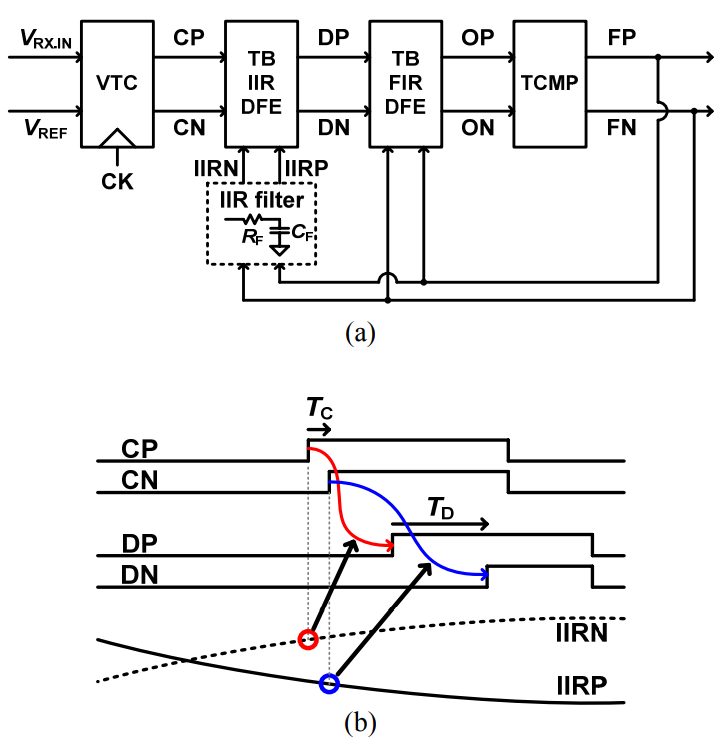Fig. 6. Mathematical model of proposed TB RX with IIR DFE and channel.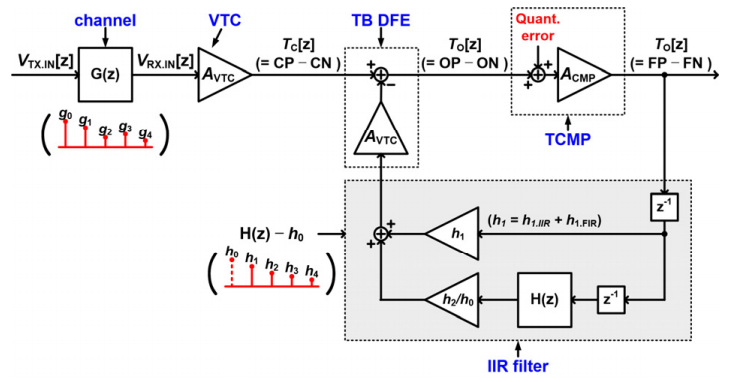where VIIR = IIRP - IIRN and AIIR is the gain of the TB IIR DFE block. VIIR is generated by an IIR filter that accepts the RZ decision data (FP, FN) as input; the IIR filter is a differential RC filter that has the same RC time constant as the channel. The IIR DFE widens the time difference TD between the clock-like signal pair (DP, DN) such that |TD| > |TC| (Fig. 5(b)). The 1-tap FIR DFE further widens the time interval between the rising edges by generating a clock-like signal pair (OP, ON) such that |TO| > |TD|; TO is the time interval from the rising edge of OP to that of ON.

The mathematical model of TB RX (Fig. 5) is summarized in Fig. 6; G(z) models the single-bit response of the real channel which connects TX and RX. H(z) is the single-bit response of a RC channel with single time constant (RC). The combination of a 1-tap IIR DFE and a 1-tap FIR DFE (shaded part of Fig. 6) generates a feedback gain of H(z) - h0; this gives the loop gain of {H(z) - h0}∙AVTC∙ACMP in Fig. 6. The forward gain is G(z)∙AVTC∙ACMP. Because both VTX.IN and VOUT have a normalized range of [-1.0 , 1.0], the VTC gain (AVTC) and the time comparator gain (ACMP) are adjusted to satisfy (9)

##### (9)
$$A_{\mathrm{VTC}} \cdot g_{0} \cdot A_{\mathrm{CMP}}=1$$

By using the loop gain, the forward gain and (9), the transfer function of the proposed transceiver can be derived as

##### (10)
$$\frac{V_{\text {OUT }}(z)}{V_{\text {TX.IN }}(z)}=\frac{g_{0}+g_{1} \cdot z^{-1}+g_{2} z^{-2}+g_{3} z^{-3}+\cdot \cdot \cdot}{g_{0}+h_{1} \cdot z^{-1}+h_{2} \cdot z^{-2}+h_{3} \cdot z^{-3}+\cdot \cdot \cdot}$$

(10) indicates that the proposed transceiver compensates the ISI of a single-time-constant channel completely.

A behavior-level simulation is presented in Fig. 7(a) to explain the operation of the proposed TB IIR DFE. TX sends a 10 Gb/s digital signal of ‘10000000111111011111 001’ (a part of PRBS-7) to RX through the channel; the channel refers to the short-reach PCB interconnect with RTX = 360 Ω, RRX = 120 Ω, L = 0.5 nH and C = 1.8 pF and 1.6 mm micro-strip line. The RX input waveform (VRX.IN) is obtained with HSPICE simulation. VRX.IN is converted into a TC signal by VTC with AVTC = 0.4 ps/mV; large ISI can be observed in VRX.IN and the TC signal. The 1-tap TB IIR DFE with the single-bit response of H(z) in Eq. (2) is used to generate a TO signal; T = 10-10 sec (10 Gb/s), and RC = 180·10-12 sec.

Fig. 7. Behavior simulation of proposed short-reach interface (a) Timing diagram of each signal node in Fig. 5, (b) Eye diagram of clock-like signals before EQ (TC) and after EQ (TO).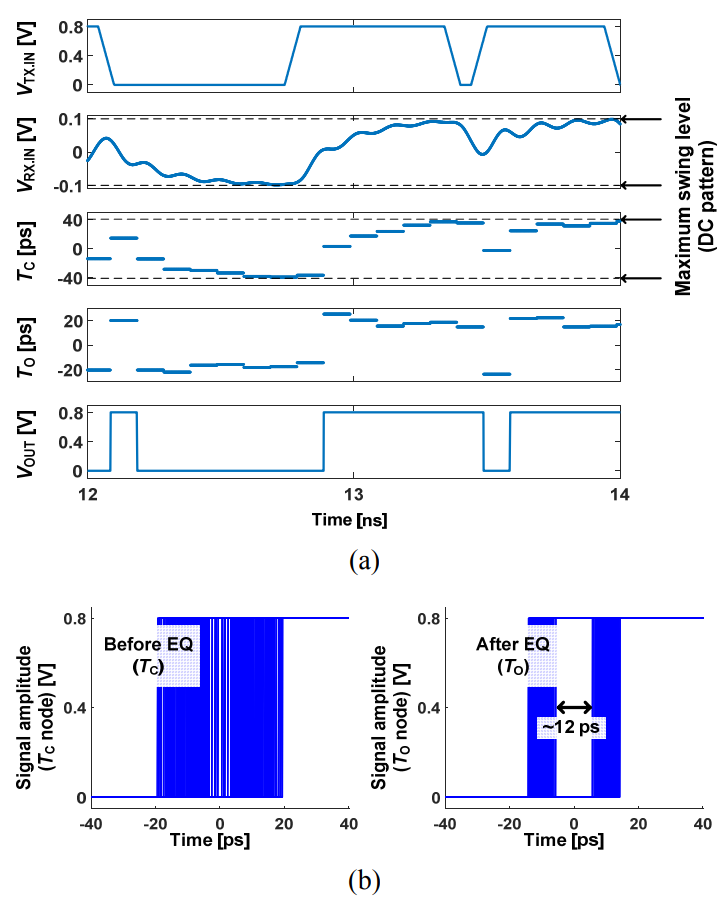In the TO signal, a clear separation into two groups can be observed (Fig. 7(a)). The effect of the IIR DFE operation can be observed more clearly in the eye patterns of the TC and TO signals (Fig. 7(b)); although eye is almost closed in the TC signal, the TO signal has a clear eye opening of 12 ps which is large enough for TCMP to separate VOUT into ‘1’ or ‘0’ signal.

## IV. CIRCUIT IMPLEMENTATION

The TB RX circuit (Fig. 6) was implemented in a quarter-rate architecture (Fig. 8) to increase the VTC gain as in the previous design (4). The same circuit is used in this work as in (4) except the IIR-DFE and the IIR-filter. A 1.6-mm micro-strip line connects TX and RX as a short-reach PCB interconnect channel. The characteristic impedance (ZO) of the micro-strip line is ~50 Ω, but a relaxed termination is used at TX and RX to save TX power (RTX, RRX > ZO); RRX is set to be one of six values (80, 96, 120, 160, 240, 480 Ω) by connecting a set of six 480-Ω resistors in parallel. A voltage-mode TX driver is used with RTX = 3·RRX. Because reducing RRX increases the maximum data-rate but it increases TX power, RRX is increased to the largest possible value at a given data rate to save power. To keep RTX = 3·RRX, RTX is implemented with a set of six 1440 Ω resistors; RTX of each TX driver is set to 1440 Ω by two analog voltages (VBP, VBN), at VRX.IN = 0.2 V, VDD = 0.8V. The same number of parallel resistors are turned on for RTX and RRX to keep RTX = 3·RRX. Four VTCs generate four clock-like signal pairs (CP0, CN0), (CP90, CN90), (CP180, CN180), (CP270, CN270) by using quarter rate clocks (CK0, CK90, CK180, CK270), respectively. Each VTC is composed of two comparators with opposite offsets ( ) as in (4); VOS = 100 mV, the linear range ranges from 50 mV to 150 mV and AVTC is 0.4 ps/mV in this work. The clock-like VTC output pairs are applied to the corresponding IIR DFE of the quarter rate architecture; the IIR DFE generates another four pairs of clock-like signals (DP0, DN0), (DP90, DN90), (DP180, DN180), (DP270, DN270) by using a differential analog signal (VIIR = IIRP - IIRN; the IIR filter output). The following FIR DFE accepts the IIR DFE output and generates another four pairs of clock-like signals (OP0, ON0), (OP90, ON90), (OP180, ON180), (OP270, ON270) by using the TCMP output pairs (FN270, FP270), (FN0, FP0), (FN90, FP90), (FN180, FP180), respectively. The FIR DFE output is applied to the corresponding TCMP input. The TCMP output pairs are also applied to the IIR filter; it generates the differential analog signal (VIIR = IIRP - IIRN) used for the IIR DFE operation.

Fig. 8. TX driver and proposed TB RX circuit in quarter rate architecture.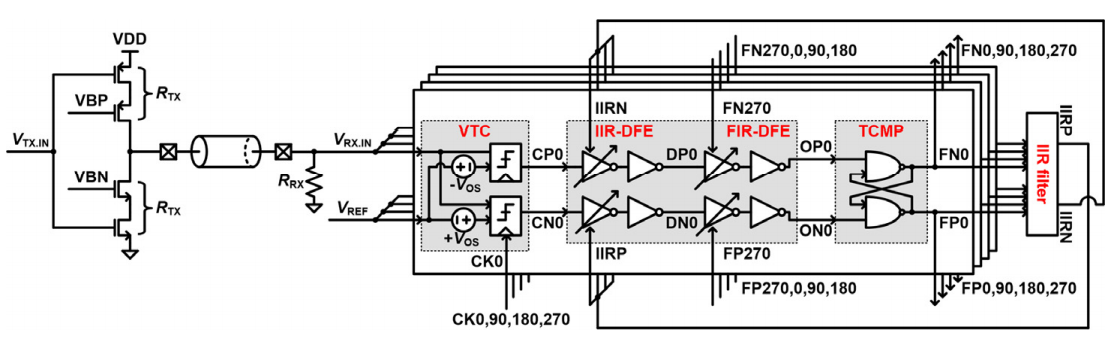The IIR filter (Fig. 9(a)) multiplexes the four pairs of the TCMP output to generate a RC-filtered differential analog signal (VIIR = IIRP - IIRN) (10). The multiplexing is required because of the infinite range of the IIR DFE operation; the equalization operation of an IIR DFE block of the quarter rate architecture is affected by all the previous decision data including the nearest three preceding data which are the TCMP outputs of other branches. The multiplexing is done by using gated NMOS input differential pairs with the quadrature signal used as the gating signal; the FP90 and FN90 signals are used for the FP0 and FN0 input signals. Because the TCMP outputs (FP0, FN0, ···) are active-low RZ signals, they are inverted to be used as the input signal of NMOS input differential pairs. The gating signals (W90, W180, W270, W0) are generated by passing the quadrature signals through AND gates; clock signals are not used for gating because the time delay from the clock sampling (falling) edge at VTC to the falling edge of TCMP output (FPn, FNn) is not constant. Due to the series connection of the differential pair and the gating transistor, the current IH or IL is injected to each branch of RF-CF low-pass filter during the conduction time interval of around 1 UI; when FPn - FNn < 0 (VRX.IN < VREF) as in Fig. 9(b), the differential output (VIIR=IIRP-IIRN) decreases with time. The conduction time interval changes from 1 UI - jitter to 1 UI + jitter; the jitter refers to the TCMP output jitter. To compensate for the channel RC time constant of around 1 ns, the RC time constant of the RF-CF filter varies up to 1.1 ns from 70 ps. RF is either 1 kΩ or 3 kΩ poly resistor and CF is a 4-b binary weighted NMOS capacitor. By adjusting IH and IL, IIRP and IIRN swings between 0.5 V and 0.7 V to match the linear input voltage range of the IIR DFE circuit.

Fig. 9. (a) Circuit diagram of proposed IIR filter, (b) Timing diagram of gating signal and differential input signal.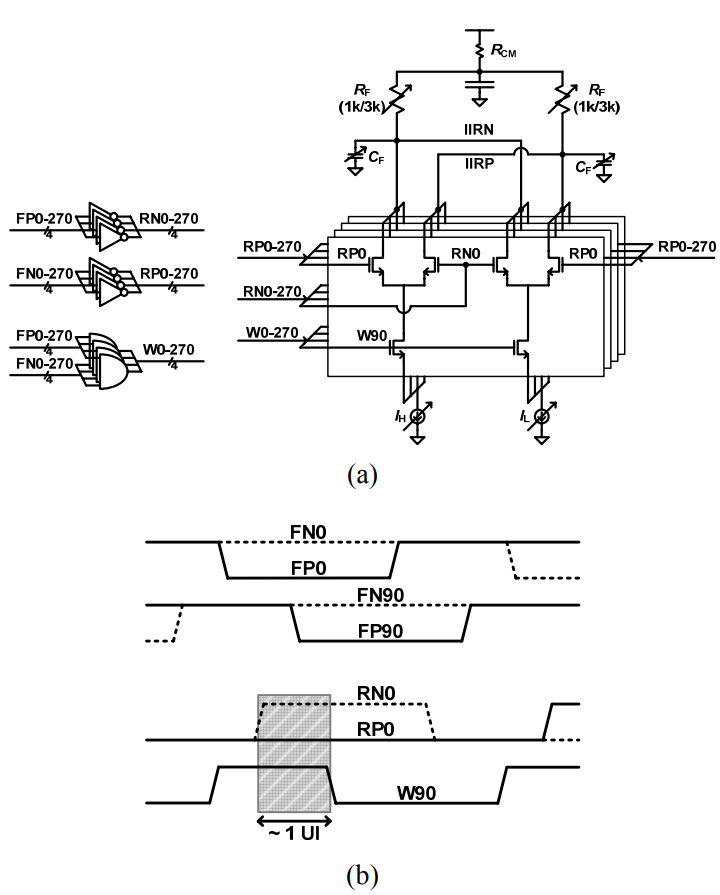The IIR DFE of Fig. 8uses current-starved inverters as in the FIR DFE (4), as shown in Fig. 10. TD (time interval from the rising edge of DP to that of DN) must be linearly proportional to the differential analog voltage VIIR as stated in Eq. (8); IIRP and IIRN swing differentially between 0.5 V and 0.7 V with the common mode voltage (VCM) of 0.6 V. By the operation of the IIR DFE circuit (Fig. 10), TD can be derived as Eq. (11).

##### (11)
$$T_{\mathrm{D}}=T_{\mathrm{C}}-\left(R_{\mathrm{ON} . \mathrm{P}}-R_{\mathrm{ON} . \mathrm{N}}\right) \cdot C_{\mathrm{M}}$$

RON.P and RON.N are the on-resistance of the NMOS M1 and M2, respectively; W/L of M1, M2 is 4 times that of the inverter NMOS. CM is the capacitance of the NMOS M3 and M4; CM decreases monotonically as VBIIR increases. RON.P - RON.N is proportional to the differential voltage VIIR (= IIRP - IIRN) for the VIIR range of [- 0.2 V, + 0.2 V] within the error bound of 2.5 %. AIIR of (8) can be derived as (12).

##### (12)
$$A_{I I R}=\frac{1}{\mu_{\mathrm{n}} C_{\mathrm{ox}} \mathrm{W}_{1} / \mathrm{L}_{1}} \cdot \frac{C_{\mathrm{M}}}{\left\{V_{\mathrm{CM}}-V_{\mathrm{TH}}+\alpha \cdot\left(\mathrm{VDD}-V_{\mathrm{TH}}\right)\right\}^{2}}$$

Fig. 11. Comparison of differential delay time between equation (lines, Eq. (11)) and simulation (symbols).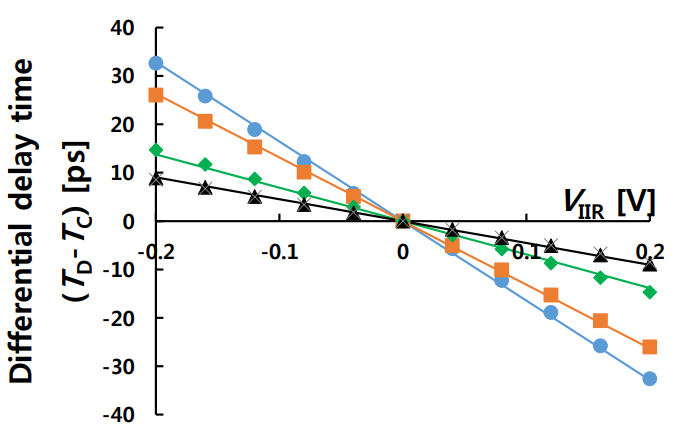α is the W/L ratio of the inverter NMOS to M1 or M2. For VBIIR from 0V to 0.8V, AIIR of Fig. 11ranges from 0.04 ps/mV to 0.16 ps/mV. VBIIR is set by a 7 bit DAC. Comparison of TD − TC between Eq. (11) (lines) and circuit simulation (symbols) demonstrates the average relative error of 4.6 % (Fig. 11). VBFIR, VBIIR and the RC time constant of the RF-CF filter are manually controlled in this work.

## V. MEASUREMENT RESULTS

The proposed TB RX was implemented in a 65-nm CMOS process (Fig. 12(a)). Chip areas of TX and RX are 1000 µm2 and 7700 µm2, respectively. The TX and RX chips are placed on PCB through COB (Fig. 12(b)); a double-bonding technique was used between a die chip pad and channel to reduce the bonding-wire inductance by half. The TX chip receives full rate data from a BER tester and transmits the data to the RX chip through a 1.6 mm micro-strip line (Fig. 12(c)). The RX returns quarter-rate data to the BER tester. The channel eye diagrams were measured by using an active probe (0.35 pF, 25 kΩ) for RRX =160 Ω, 120 Ω, 96 Ω (Fig. 13); smaller RRX gives larger eye opening.

Fig. 12. (a) Chip micrograph, (b) PCB photograph, (c) Measurement setup.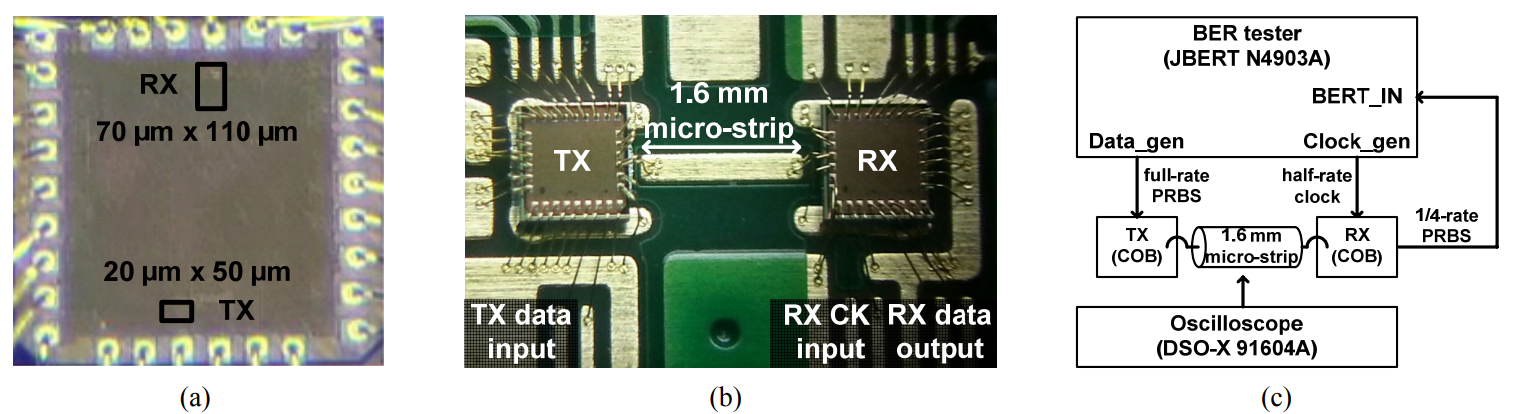Fig. 13. Measured eye diagrams at 8 Gb/s (a) RRX = 160 Ω, (b) RRX = 120 Ω, (c) RRX = 96 Ω.Fig. 14. Measured maximum data-rate vs. 1/RRX with (red circle) and without (blue X) IIR DFE. VTC linearity limit (dotted line), and RX sensitivity limit (broken line) are derived from Eq. (6), (7) with simulated single-bit responses. VDD = 0.8 V.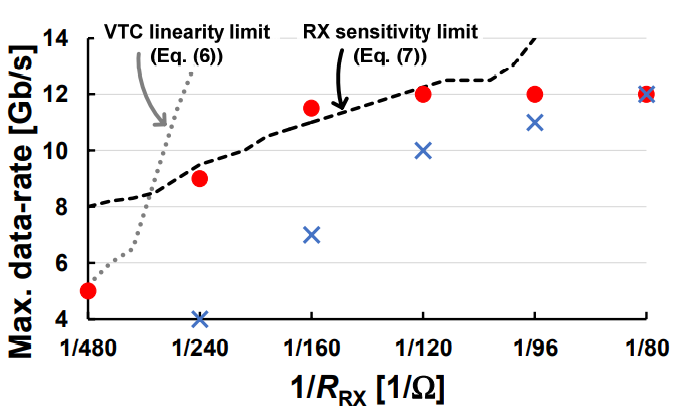Fig. 15. Measured energy efficiency of proposed TX and RX circuits vs. 1/RRX; VDD = 0.8 V (red circles), VDD = 0.75 V (blue X).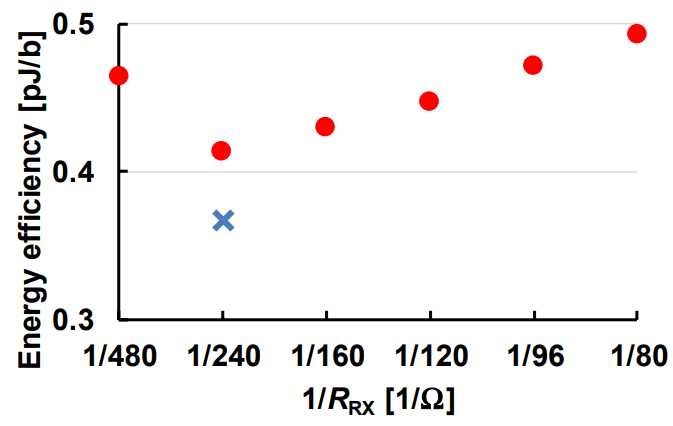A BER measurement demonstrated a large increase of maximum data rate (BER < 10-12) by the 1-tap IIR DFE for the entire range of RRX (Fig. 14) at VDD = 0.8 V; red circles (O) are the measured highest data-rate achieved with the 1-tap IIR DFE and blue crosses (X) are those without equalization. As discussed in Section II, the measured maximum data-rate with 1-tap IIR DFE are limited by either the VTC linearity limit (Eq. (6)) or the RX sensitivity limit (Eq. (7)). The measured data rate was limited to 12 Gb/s because of the BER tester limit. The minimum energy efficiency of 0.367 pJ/b was achieved at RRX = 240 Ω, data-rate = 8 Gb/s and VDD = 0.75 V. The maximum data-rate of 12 Gb/s was achieved at RRX = 120 Ω; the energy efficiency was 0.446 pJ/b. The energy efficiency ranges from 0.367 pJ/b to 0.49 pJ/b (Fig. 15); the six red circles are the same as those in Fig. 14with VDD = 0.8 V.

The measured bathtub curves demonstrate the successful operation of the IIR DFE at RRX = 240 Ω and 8 Gb/s (Fig. 17(a)) and RRX = 120 Ω and 12 Gb/s (Fig. 17(b)). With the help of relaxed termination, the power consumption of TX (pre-driver + main-driver) is greatly reduced to 23 % of the total transceiver power (Fig. 16); the TX power is reduced to 44 % of the 50-Ω terminated TX (5).

The proposed TB transceiver is compared with the previous works with the same technology node of 65 nm (Table 1). The TX power is 37 % of the best previous value.

Fig. 17. Measured bathtub curves (a) RRX = 240 Ω, data-rate = 8 Gb/s and VDD = 0.75 V, (b) RRX = 120 Ω, data-rate = 12 Gb/s and VDD = 0.8 V.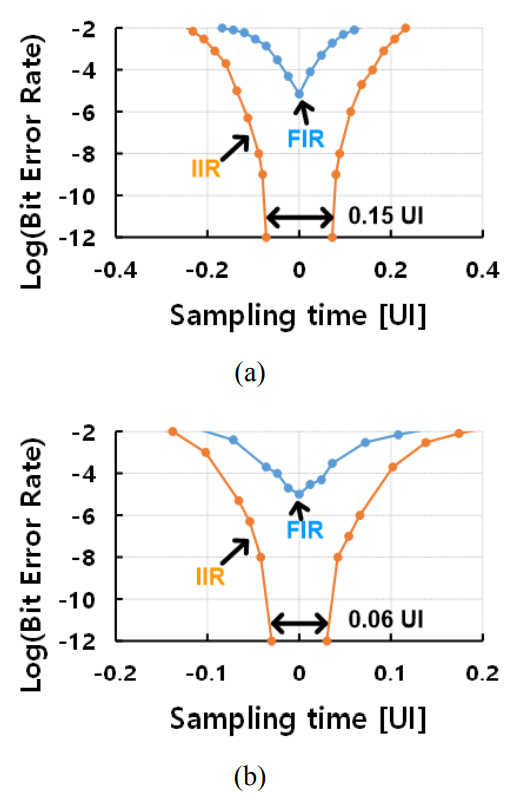Table 1. Performance comparison of low-power transceivers in 65 nm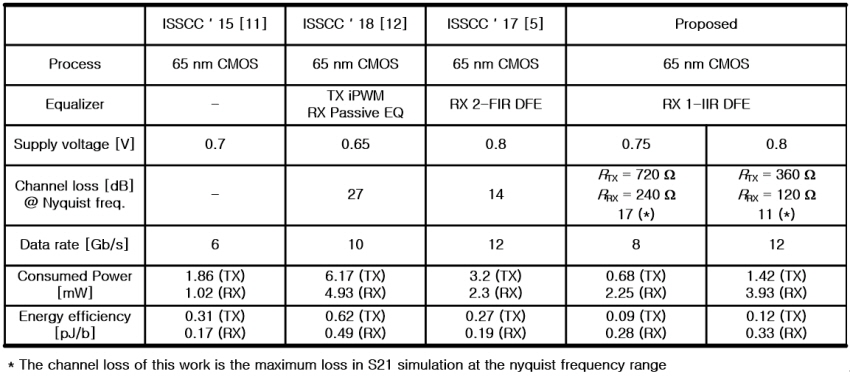## VI. CONCLUSIONS

To reduce the energy efficiency of time-based transceiver with short-reach PCB interconnect, the RX termination resistance was increased to 120 Ω or 240 Ω with the TX termination resistance being three times the RX termination resistance. A voltage mode driver is used at TX for low power. A 1-tap IIR DFE was used at RX to compensate for the increased channel ISI due to the increased termination resistance. A 1.6 mm micro-strip transmission line was modeled as a RC channel with single time constant because it is shorter than the critical length for transmission line analysis, which is 3.2 mm for a pulse signal with the 10 %-to-90 % rise time of 60 ps. The 1-tap IIR DFE was added to the time-based transceiver with a 1-tap FIR at RX (4); The proposed time-based transceiver chip fabricated in a 65 nm CMOS process achieved the minimum energy efficiency of 0.367 pJ/b with RX termination resistance of 240 Ω at 8 Gb/s, and the maximum data rate of 12 Gb/s and the energy efficiency of 0.446 pJ/b and RX termination resistance of 120 Ω. The TX and RX chip areas are 1000 µm2 and 7700 µm2, respectively.

### ACKNOWLEDGMENTS

This work was supported in part by Institute of Information & Communications Technology Planning & Evaluation (IITP) Grant funded by the Ministry of Science and ICT (MSIT), Korea (No. 2019001394, Automatic Design Generation of Ultra-High-Speed I/O Circuit to support Intelligent Semiconductor Devices) and in part by Samsung Electronics.

### REFERENCES

1
Cho J. H., Kim J., Lee W. Y., Lee D. U., Kim T. K., Park H. B., Jeong C., Park M.-J., Baek S. G., Choi S., Yoon B. K., Choi Y. J., Lee K. Y., Shim D., Oh J., Kim J., Lee S.-H., Feb 2018, A 1.2V 64Gb 341GB/s HBM2 Stacked DRAM with Spiral Point-to-Point TSV Structure and Improved Bank Group Data Control, in ISSCC Dig. Tech. Papers, pp. 208-2092
Tsai M., Chiu R., He E., Chen J. Y., Chen R., Tsai J., Wang Y.-P., 2018, Innovative Packaging Solutions of 3D System in Package with Antenna Integration for IoT and 5G Application, in Proc. 20th Electronics Packaging Technology Conf. (EPTC)3
Bogatin E., , Signal Integrity Simplified, Prentice Hall Modern Semiconductor Design Series.4
Yi I.-M., Chae M.-K., Hyun S.-H., Bae S.-J., Choi J.-H., Jang S.-J., Kim B., Sim J.-Y., Park H.-J., Jun 2018, A time-based receiver with 2-tap decision feedback equalizer for single-ended mobile DRAM interface, IEEE J. Solid-State Circuits, Vol. 53, No. 1, pp. 144-1545
Yi I.-M., Chae M.-K., Hyun S.-H., Bae S.-J., Choi J.-H., Jang S.-J., Kim B., Sim J.-Y., Park H.-J., Feb 2017, A time-based receiver with 2-tap DFE for a 12Gb/s/pin single-ended transceiver of mobile DRAM interface in 0.8V 65 nm CMOS, in IEEE Int. Solid-State Circuits Conf. (ISSCC) Dig. Tech. Papers, pp. 400-4016
Chiu P.-W., Kundu S., Tang Q., Kim C. H., 2017, A 10Gb/s 10mm On-Chip Serial Link in 65nm CMOS Featuring a Half-Rate Time-Based Decision Feedback Equalizer, in IEEE Symposium on VLSI Circuits (VLSIC), pp. 56-577
Oh T., Harjani R., 2014, High Performance Multi-Channel High-Speed I/O Circuits, 1st ed. springer, pp. 1-98
Shahramian S., Chan Carusone A., Jul 2015, A 0.41 pJ/bit 10 Gb/s hybrid 2 IIR and 1 discrete-time DFE tap in 28 nm-LP CMOS, IEEE J. Solid-State Circuits, Vol. 50, No. 7, pp. 1722-17359
Shahramian S., Dehlaghi B., Carusone A. C., Dec 2016, Edge-based adaptation for a 1 IIR + 1 discrete-time tap DFE converging in 5 µs, IEEE J. Solid-State Circuits, Vol. 51, No. 12, pp. 3192-320310
Kim B., Liu Y., Dickson T. O., Bulzacchelli J. F., Friedman D. J., Dec 2009, A 10-Gb/s compact low-power serial I/O with DFE-IIR equalization in 65-nm CMOS, IEEE J. Solid-State Circuits, Vol. 44, No. 12, pp. 3526-353811
Choi W.-S., Shu G., Talegaonkar M., Liu Y., Wei D., Hanumolu L. Benini and P. K., Feb 2015, A 0.45-to-0.7V 1-to-6 Gb/s 0.29-to-0.58 pJ/b source-synchronous transceiver using automatic phase calibration in 65 nm CMOS, in IEEE ISSCC Dig. Tech. Papers, pp. 66-6712
Ramachandran A., Anand T., Feb 2018, A 0.5-to-0.9V, 3-to-16Gb/s, 1.6-to3.1pJ/b wireline transceiver equalizing 27dB loss at 10Gb/s with clock-domain encoding using integrated pulse-width modulation (IPWM) in 65nm CMOS, in IEEE ISSCC Dig. Tech. Papers., pp. 268-270## Author

Min-Kyun Chae received the B.S. and M.S. degrees in electronic and electrical engineering from the Pohang University of Science and Technology (POSTECH), Pohang, South Korea, in 2012 and 2014, respectively, where he is currently pursuing the Ph.D. degree in electronic and electrical engineering.

His current research interests include high-speed low-power I/O circuits.

Seung-Jun Bae received the B.S. and Ph.D. degrees in electrical engineering from the Pohang University of Science and Technology (POSTECH), Pohang, South Korea, in 2000 and 2005, respectively.

In 2005, he joined Samsung Electronics, Hwaseong, South Korea, where he was involved in the design of high-bandwidth DRAM such as GDDR5, LPDDR4/4X, DDR4, HBM2, and GDDR6.

From 2013 to 2014, he was a Visiting Scientist with the Massachusetts Institute of Technology (MIT), Cambridge, MA, USA.

He is currently a Vice President of the Mobile/Graphic DRAM Design Group.

His current research interests include high-speed interface circuits, signal/power integrity, high-speed analog-to-digital converters, and next-generation memory architecture.

Dr. Bae has served on the Technical Program Committees of the IEEE International Solid-State Circuits Conference (ISSCC) from 2016.

Jung-Hwan Choi was born in Daegu, South Korea, in 1968.

He received the B.S. degree in electrical engi-neering from Kyungpook National University, Daegu, in 1990, and the M.S. and Ph.D. degrees in electrical engineering from the Korea Advanced Institute of Science and Technology, Daejeon, South Korea, in 1992 and 1997, respectively.

In 1997, he joined Samsung Electronics, Hwaseong, South Korea, where he was involved in the design of Rambus, XDR DRAM, and high-speed I/O interface for memory applications.

He is a currently a Master with Samsung Electronics, where he is responsible for the design of DRAM interface and the development of high-speed DRAM interfaces for the next generation, including LPDDRx and DDRx.

His current research interests include the design of monolithic microwave IC, high-speed memory, and high-frequency measurement.

Kwang-Il Park received the B.S., M.S., and Ph.D. degrees in electrical and electronic engineering from the Korea Advanced Institute of Science and Technology (KAIST), Daejeon, South Korea, in 1993, 1995, and 1999, respectively.

He joined LG Semicon Corporation Ltd., Seoul, South Korea, in 1999, where he was involved in the Rambus DRAM and PLL.

Since 2003, he has been with Samsung Electronics, Hwaseong, South Korea.

He is currently a Senior Vice President with the DRAM Design Division.

His current research interests include high-speed, high-density, and low-power DRAM and interface design.

Jung-Bae Lee was born in Seoul, South Korea, in 1967.

He received the B.S., M.S., and Ph.D. degrees in electronics engineering from Seoul National University, Seoul, in 1989, 1991, and 1995, respectively.

He joined the DRAM Design Team, Samsung Electronics, Hwaseong, South Korea, in 1995, as a Circuit Design Engineer, where he participated in the development of various DRAM products, including DDR, DDR2, DDR3, GDDR, LPDDR2, and LPDDR3.

He became the Head of the DRAM Design Team in 2012, the Memory Product Planning and Application Engineering Team in 2014, and Quality Assurance in 2017.

His leadership through various backgrounds, including design, product planning, and quality assurance enhance overall completeness of Samsung memory products.

Since 2019, he has been leading DRAM product and technology.

His research interests include the design of high-speed low-power architecture for the next-generation memory and noise phenomena in devices.

Hong-June Park (M’88-SM’13) received the B.S. degree in electronic engineering from Seoul National University, Seoul, South Korea, in 1979, the M.S. degree from the Korea Advanced Institute of Science and Technology, Daejeon, South Korea, in 1981, and the Ph.D. degree in electrical engineering and computer sciences from the University of California, Berkeley, CA, USA, in 1989.

From 1981 to 1984, he was a CAD engineer with ETRI, Daejeon.

From 1989 to 1991, he was a Senior Engineer with the TCAD Department of INTEL, USA.

In 1991, he joined the Electronic and Electrical Engineering Department as a Faculty Member, Pohang University of Science and Technology, Pohang, South Korea, where he is currently a Professor.

His current research interests include CMOS analog circuit design such as high-speed interface circuits, ROIC of touch sensors, and analog/digital beamformer circuits for ultrasound medical imaging.

Prof. Park is a member of IEEK.

He served as the Editor-in-Chief of the Journal of Semiconductor Technology and Science, an SCIE journal from 2009 to 2012, as the Vice President of IEEK in 2012, and as a Technical Program Committee Member of ISSCC, SOVC, and A-SSCC for several years.